# RD Sharma Solutions Class 9 Chapter 7 Introduction To Euclids Geometry

Read RD Sharma Solutions Class 9 Chapter 7 Introduction To Euclids Geometry below, students should study RD Sharma class 9 Mathematics available on Studiestoday.com with solved questions and answers. These chapter wise answers for class 9 Mathematics have been prepared by teacher of Grade 9. These RD Sharma class 9 Solutions have been designed as per the latest NCERT syllabus for class 9 and if practiced thoroughly can help you to score good marks in standard 9 Mathematics class tests and examinations

Question 1.  Define the following terms:
(i) Line segment
(ii) Collinear points
(iii) Parallel lines
(iv) Intersecting lines
(v) Concurrent lines
(vi) Ray
(vii) Half-line
Solution 1.
(i) Line segment:- The line which has two end points it is called line segment. Here (ab)  is a line segment.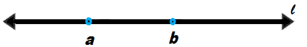(ii) Collinear points:- When two or more point lie on a same line. Here point a, b and c are collinear points.(iii) Parallel lines:- Two lines having no common points are parallel lines. Two or more than two lines are parallel if they have no intersecting point. Both lines have common distance from each other. For example green and orange lines are parallel to each other.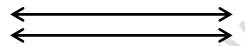(iv) Intersecting lines:- Two lines are intersecting line if they have a common point. The common point is called point of intersection. Here point p is a intersecting point.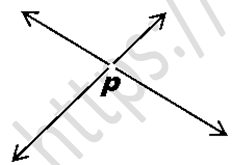(v) Concurrent lines:- Three or more than three lines are said to ne concurrent if there is a point which lies on all of them.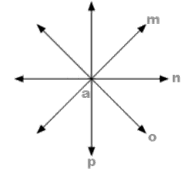(vi) Ray:- A line which one end point is fixed and the part can be extended endlessly. In other words A ray is defined as the part of the line with one end point such that it can be extended infinitely in the other direction. For example here (ab).(vii) Half-line:- If A, B, C be the points on a line, such that b lies between a and c, and if we remove the point b from line, the other two parts of that remain are each corner called half-line. It is different from ray as end point is not included in the half-line.Question 2.
(i) How many lines can pass through a given point?
(ii) In how many points can two distinct lines at the most intersect?
Solution 2.
(i) Infinitely many lines can pass through a given point.(ii) Two lines can intersect from only one point.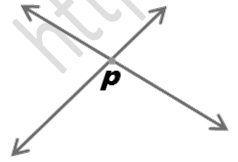Question 3.
(i) Given two points P and Question , find how many line segments do they deter-mine.
(ii) Name the line segments determined by the three collinear points P, Q and R.
Solution 3.
(i) One line segment we can determine.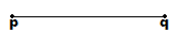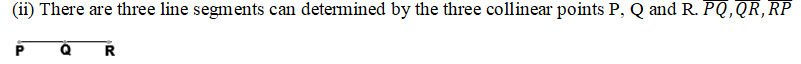Question 4. Write the turth value (T/F) of each of the following statements:
(i) Two lines intersect in a point.
(ii) Two lines may intersect in two points.
(iii) A segment has no length.
(iv) Two distinct points always determine a line.
(v) Every ray has a finite length.
(vi) A ray has one end-point only.
(vii) A segment has one end-point only.
(viii) The ray AB is same as ray BA.
(ix) Only a single line may pass through a given point.
(x) Two lines are coincident if they have only one point in common.
Solution 4.
(i) False, it is not compulsory that the two lines always intersect each other. For example parallel lines not intersect.
(ii) False, as there is only one common point between two intersecting lines.
(iii) False, a segment is a part of a line so it is always of a definite length.
(iv) True, a line can be determined by minimum of two distinct points.
(v) False, a ray has not a finite length as it can be stretched infinitely.
(vi) True, there is only a one end point in a ray other end will stretched infinitely.
(vii) False, a line segment is a defined part and it has a definite length.
(viii) False, as only one point is defined in a ray other is stretched infinitely so we can’t denote the ray with reversing name.
(ix) False, many lines can pass through a given point.
(x) False, two lines can said coincident if they lie exactly on each other.

Question 5. In Fig. 7.17, name the following:(i) five line segments.
(ii) five rays
(iii) four collinear points
(iv) two pairs of non-intersecting line segments.
Solution 5.(iii) Four collinear points are in the given figure:- C,D,Q,S
(iv) Two pairs of non-intersecting line segments are:- AB and CD, AB and LS

Question 6. Fill in the blanks so as to make the following statements true:
(i) Two distinct points in a plane determine a _________line.
(ii) Two distinct _________ in a plane cannot have more than one point in common.
(iii) Given a line and a point, not on the line, there is one and only _________line which passes through the given point and is _________to the given line.
(iv) A line separates a plane into________parts namely the ________and the________itself.
Solution 6.
(i) Two distinct points in a plane determine a  unique  line.
(ii) Two distinct  lines  in a plane cannot have more than one point in common.
(iii) Given a line and a point, not on the line, there is one and only   perpendicular  line which passes through the given point and is   perpendicular   to the given line.
(iv) A line separates a plane into  three  parts namely the  two half plane  and the  line   itself.